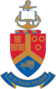• MIT804 - Introduction to Mathematical Optimisation for Big Data Science

### MIT804 - Introduction to Mathematical Optimisation for Big Data Science

• ###### 5 CREDITS

Prerequisites:None

In this module students will be introduced to Mathematical Optimization through gaining knowledge about the theory and algorithms to solve optimisation problems. Topics will include: Linear programming, unconstrained optimization, equality constrained optimization, general linearly and nonlinearly constrained optimization, quadratic programming, global optimization, Theory and algorithms to solve these problems.

##### Course Coordinators